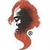0
Beantwortet

# Scatter series points with different color

Xavier Hahn vor 6 Jahren in API aktualisiert von anonymous vor 6 Jahren
I'm trying to make a graph with a few points, with some that should be a different color based on whether they are "outliers" or not. Basically, I display scatter points, and if a property on the list I'm binding the ScatterSeries to is "true", then the color of the point should be red, otherwise it should be black.

I've tried using the `LinearColorAxis` for this, but no luck yet. Here's the code (WPF) I have now:
```<oxy:plot title="Repetitions">
<oxy:plot.axes>
<oxy:linearcoloraxis key="Outliers" position="Right" minimum="0" maximum="1" highcolor="Red" lowcolor="Black"></oxy:linearcoloraxis>
<oxy:linearaxis position="Bottom" title="Measurement"></oxy:linearaxis>
<oxy:plot.series>
<oxy:scatterseries itemssource="{Binding Item.Repetitions}" coloraxiskey="Outliers" datafieldx="RepetitionNbr" datafieldy="ExcessRayleighRatio" datafieldvalue="IsOutlierValue"></oxy:scatterseries>
</oxy:plot.series>
</oxy:plot.axes>
</oxy:plot>
```
The DataFieldValue does seem to be took (if I put a breakpoint in the property, I can see the binding working, the IsOutlierValue is a "double" property that returns 1.0 if I want the point "red", and 0.0 if I want it black. But the points don't change color.Wird überprüft
Try changing `Minimum=0.1` and `Maximum=0.9` in your `LinearColorAxis`
The `HighColor` is used for values above the `Maximum` defined in the axis!And set `Position = None` if you don't need to see the color axis.+1
```        [Example("Colored outliers")]
public static PlotModel ColoredOutliers()
{
var plotModel1 = new PlotModel { Title = "Colored outliers" };
plotModel1.Axes.Add(new LinearColorAxis { Position = AxisPosition.None, Minimum = 0.1, Maximum = 0.9, HighColor = OxyColors.Red, LowColor = OxyColors.Black });
var series = new ScatterSeries();
series.Points.Add(new ScatterPoint(0, 0) { Value = 0 });
series.Points.Add(new ScatterPoint(1, 0) { Value = 0 });
series.Points.Add(new ScatterPoint(2, 1) { Value = 1 });
series.Points.Add(new ScatterPoint(3, -1) { Value = 1 });